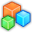# Microsoft Small BasicProgram Listing: SWQ334
' Leaf
' Version 0.1
' Last update 2018-12-23

GraphicsWindow.Title = "Leaf | Toggle Shapes opacity with mouse"
gw = 598
gh = 428
GraphicsWindow.Width = gw
GraphicsWindow.Height = gh
op = 100
pw = 4
x = gw / 2
y = gh / 2
GraphicsWindow.PenColor = "LightGray"
GraphicsWindow.DrawLine(0, y, gw, y)
GraphicsWindow.DrawLine(x, 0, x, gh)
GraphicsWindow.MouseDown = OnMouseDown
While "True"
If bottom Then
width = 150
height = 200
stem = 50
pc = "Black"
bc = "YellowGreen"
top = "True"
bottom = "False"
center = "True"
Leaf()
Else
width = 100
height = 150
stem = 50
pc = "Black"
bc = "Green"
top = "True"
bottom = "True"
center = "True"
Leaf()
EndIf
Delay()
RemoveShapes()
EndWhile

i = i + 1
GraphicsWindow.PenColor = pc
GraphicsWindow.PenWidth = pw
GraphicsWindow.BrushColor = bc
Shapes.Move(shp[i], x, y)
Shapes.SetOpacity(shp[i], op)
EndSub

If 0 < pw Then
i = i + 1
GraphicsWindow.PenColor = pc
GraphicsWindow.PenWidth = pw
shp[i] = Shapes.AddLine(x1, y1, x2, y2)
Shapes.Move(shp[i], x, y)
Shapes.SetOpacity(shp[i], op)
EndIf
EndSub

i = i + 1
GraphicsWindow.PenColor = pc
GraphicsWindow.PenWidth = pw
GraphicsWindow.BrushColor = bc
shp[i] = Shapes.AddTriangle(x1, y1, x2, y2, x3, y3)
Shapes.Move(shp[i], x, y)
Shapes.Rotate(shp[i], angle)
Shapes.SetOpacity(shp[i], op)
EndSub

Sub Delay
t = Clock.ElapsedMilliseconds
mouseDown = "False"
While Clock.ElapsedMilliseconds < (t + 3000)
If mouseDown Then
If op = 100 Then
op = 70
Else
op = 100
EndIF
For i = 1 To Array.GetItemCount(shp)
Shapes.SetOpacity(shp[i], op)
EndFor
mouseDown = "False"
EndIf
EndWhile
EndSub

Sub Leaf
' param width - the width of the leaf
' param height - the height of the ellipse in the leaf
' param stem - the length of the stem
' param pc - pen color for the border, the midvein and the stem
' param bc - brush color for the leaf
' param top - "True" if the top angle needed
' param bottom - "True" if the bottm angle needed
' param center = "True" if the midvein needed
sqr2 = Math.SquareRoot(2)
tw = width / sqr2
th = height / sqr2 / 2
_bc = bc
_pc = pc
_pw = pw
_x = x
_y = y
x = _x - width / 2
y = _y - height / 2
i = 0
x1 = tw / 2
y1 = 0
x2 = 0
y2 = th
x3 = tw
y3 = th
s = Math.SquareRoot(width * width + height * height)
w = pw * width / s
h = pw * height / s
If top Then
pw = 0
angle = 0
x = _x - tw / 2
y = _y - th * 2
ty = y + h
pw = _pw
y1 = h
x2 = w / 2
y2 = th + h / 2
x2 = tw - w / 2
bc = _pc
pw = 0
x1 = w
y1 = 0
x2 = 0
y2 = h
x3 = 2 * w
y3 = h
x = _x - w
Else
ty = _y - height / 2 + pw / 2
EndIf
bc = _bc
x1 = tw / 2
y1 = 0
x2 = 0
y2 = th
x3 = tw
y3 = th
If bottom Then
angle = 180
x = _x - tw / 2
y = _y + th
pw = 0
angle = 0
y1 = th - h
x2 = w / 2
y2 = -h / 2
pw = _pw
x2 = tw - w / 2
y2 = -h / 2
angle = 180
pw = 0
bc = _pc
x1 = w
y1 = 0
x2 = 0
y2 = h
x3 = 2 * w
y3 = h
x = _x - w
by = _y + 2 * th - h
y = by
Else
by = _y + height / 2 - pw / 2
EndIf
pw = _pw
angle = 0
If center Or (0 < stem) Then
x = _x
If center Then
y = ty
Else
y = by
EndIf
x1 = 0
y1 = 0
x2 = 0
If center Then
y2 = by - ty + stem
Else
y2 = stem
EndIf
EndIf
x = _x
y = _y
EndSub

Sub OnMouseDown
mouseDown = "True"
EndSub

Sub RemoveShapes
n = Array.GetItemCount(shp)
For i = 1 To n
Shapes.Remove(shp[i])
shp[i] = ""
EndFor
EndSub